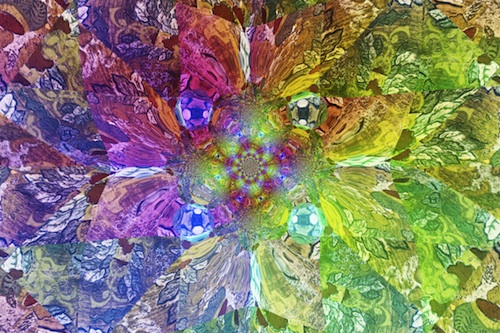# Algorithmic Movie (with texture)

We would like to share a new algorithmic movie we have created.

Since the mid 90’s we have been dabbling off and on with a combination of algorithmic and genetic art (see: What is “Genetic Art?” or try running the Java code directly in your browser). Every once in a while we return to the project and generate something we would like to share.

For this project we have used formulas over the variables “x” and “y” to describe how color varies as a function of position on our canvas.

This has allowed formulas like:

( + ( mod ( iexp k ) ( isin ( / j ( / ( x + i y + j x + k y ) k ) ) ) ) ( mod ( iexp k ) ( isin ( / j ( / ( x + i y + j x + k y ) k ) ) ) ) )

To generate pictures like this:We then add a source-texture from C. Estrade’s “Full-Color Japanese Textile Designs CD-ROM and Book” (Dover, unrestricted use):Which (with a slightly modified formula) yields a picture like this:

( + ( subst ( mod ( iexp k ) ( isin ( / j ( / ( x + i y + j x + k y ) k ) ) ) ) Img23 ) ( mod ( iexp k ) ( isin ( / j ( / ( x + i y + j x + k y ) k ) ) ) ) )We can further modify the formula to depend on time (represented by the new variable “z”):

( + ( subst ( mod ( iexp k ) ( isin ( / j ( / ( x + i y + j (x +z) + k (y + z) ) k ) ) ) ) Img23 ) ( mod ( iexp k ) ( isin ( / j ( / ( x + i y + j (x +z) + k (y + z) ) k ) ) ) ) )

And get a movie like this:

What we have previously called “genetic art” was the system of automatically combining and re-combining fragments of formulas using user votes and preferences (so nobody would have to see or understand these ugly formulas to produce art). What we now present is a larger “algebra” of “simple picture plus pattern = complicated pictures” and “picture plus time transformations = movie.”

Categories: Mathematics

Tagged as:### jmount

Data Scientist and trainer at Win Vector LLC. One of the authors of Practical Data Science with R.